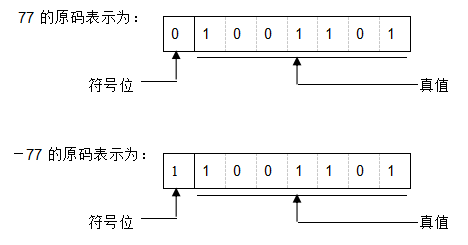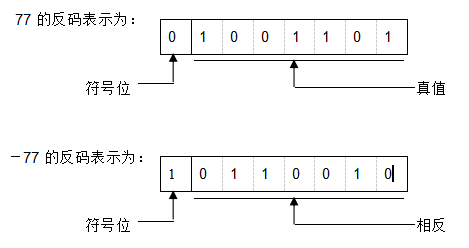# CSP 初赛数据结构与算法复习

17 October 2019 | duanyll | Tags: 数据结构

## 数制转换

K进制转10进制: 按权展开, 小数点左边第$i$位的权为$K^{i-1}$, 右边第$i$位的权为$K^{-i}$.

10进制转K进制: 整数部分除K取余, 小数部分乘K取整.

## 栈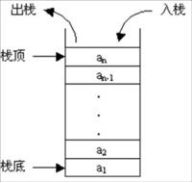## 二叉树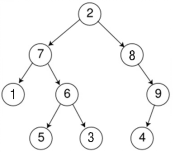n个点可以构造的不同二叉树种类数: Catalan 数

### 二叉树的性质

1. 二叉树第$i$层上至多有$2^{i-1}$个节点.
2. 深度为$k$的二叉树至多有$2^k-1$个节点, 至少有$k$个节点.
3. 二叉树中, 叶子节点个数为$n_0$, 度为2的节点个数为$n_2$, 则$n_0 = n_2 + 1$.
• 推广: K叉树中, 叶子节点个数为$n_0$, 度为K的节点个数为$n_k$, 则
$n_0 = \sum((k-1)n_k)+1$

### 完全二叉树和满二叉树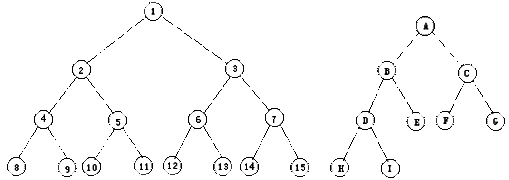1. 满二叉树 $\subset$ 完全二叉树
2. 在满二叉树的最下层上, 从最右边开始连续删去若干结点后得到的二叉树仍然是一棵完全二叉树.
3. 完全二叉树上, 若一个节点没有左孩子, 则他一定没有右孩子.
4. 有$n$个节点的完全二叉树深度为$\lfloor \log_2n\rfloor + 1$

### 堆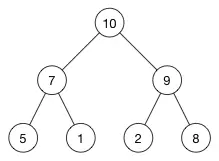• 堆总是一棵完全二叉树.
• 堆中某个节点的值总是不大于或不小于其父节点的值.

STL中, 可以用以下函数维护一个位于数组上的堆.

make_heap(first, last, cmp);
push_heap(first, last, cmp);
pop_heap(first, last, cmp);
sort_heap(first, last, cmp);


## 排序算法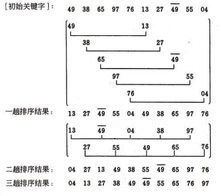## 图论算法

• 最小生成树
• Kruskal: 按边权排序, 每次取最小.
• Prim: 每个点出边按边权排序, 取链接不同连通块最小
• 最短路
• Floyd
• Dijkstra
• SPFA
• 拓扑排序

## 计算机中数的表示